>  CHEMISTRY TEST 4

# CHEMISTRY TEST 4

Test Description

## 30 Questions MCQ Test | CHEMISTRY TEST 4

 1 Crore+ students have signed up on EduRev. Have you?
CHEMISTRY TEST 4 - Question 1

### Buna-N synthetic rubber is a copolymer of

CHEMISTRY TEST 4 - Question 2

### Which of the following statements is not correct ?

CHEMISTRY TEST 4 - Question 3

### Which of the following has angular node but no spherical node ?

CHEMISTRY TEST 4 - Question 4

Which is the correct order of bond lengths x, y and z in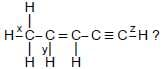CHEMISTRY TEST 4 - Question 5

Which of the following is correct for the reaction NH3 (g) + HCl (g) → NH4Cl(s)?

CHEMISTRY TEST 4 - Question 6

One gram of a solute PQ2 present in 52 g water gives ΔTb as 0.156 K and one gram of PQ3 in 52 g water gives ΔTf as 0.125. At. wt. of P and Q are

CHEMISTRY TEST 4 - Question 7

The conjugate base of [Al(H2O)3(OH)3] is

CHEMISTRY TEST 4 - Question 8

Which of the following statements is not correct ?

CHEMISTRY TEST 4 - Question 9

In which of the following sets does the oxygen atom has oxidation number in increasing order ?

CHEMISTRY TEST 4 - Question 10

For the given three cells, which of the following is correct ?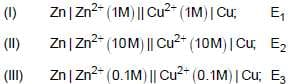CHEMISTRY TEST 4 - Question 11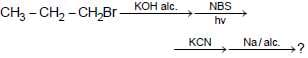The product is

CHEMISTRY TEST 4 - Question 12

Which of the following is correct w.r.t. lyophobic colloids ?

CHEMISTRY TEST 4 - Question 13

Acetophenone when reacted with a base, C2H5ONa yields a stable compound which has the structure

CHEMISTRY TEST 4 - Question 14

What is the expected pH of 0.01 M CH3COOH ?

CHEMISTRY TEST 4 - Question 15

A gaseous mixture contains CO2 and H2O (vapour) in the ratio 4 : 3 by mass. The ratio of the number of molecules is

CHEMISTRY TEST 4 - Question 16

Which of the following is/are colourless ?
I. SrCl3 ; II. TiCl4 ; III. Cu2Cl2 ; IV. ZnCl2 ?

CHEMISTRY TEST 4 - Question 17

Nickel (Z = 28) combines with uninegative monodentate ligand X- to form a paramagnetic complex (NiX4)2-. The number of unpaired electron (s) in the nickel and geometry of this complex ion are, respectively

CHEMISTRY TEST 4 - Question 18

The reducing power of divalent species in 14th group increases as

CHEMISTRY TEST 4 - Question 19

Which of the following shows wrong matching ?

CHEMISTRY TEST 4 - Question 20

Which of the following 1 M aqueous solution will have highest pH ?

CHEMISTRY TEST 4 - Question 21

Which of the following can not be used for the estimation of nitrogen in it by Kjeldahl’s method ?

CHEMISTRY TEST 4 - Question 22

The IUPAC nomenclature of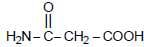CHEMISTRY TEST 4 - Question 23

Which of the foolowing is correct statement ?

CHEMISTRY TEST 4 - Question 24

The value of compressibility factor at the critical state of a van der Waals gas is

CHEMISTRY TEST 4 - Question 25

The correct order of reactivity of under mentioned alkenes for reaction with HBr is

CHEMISTRY TEST 4 - Question 26

For the reaction,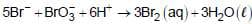.if the rate of disappearance of Br- is 2.5 x 10-2 mol L-1 s-1, the rate of appearance of Br2 will be

CHEMISTRY TEST 4 - Question 27

Which of the following shows the correct decreasing order of acidic nature ?

CHEMISTRY TEST 4 - Question 28

Which of the following order is correct w.r.t. the radius ?

CHEMISTRY TEST 4 - Question 29

From the adjacent graphs of oxide formation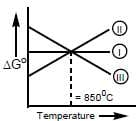I. 2Fe + 3O2 → 2Fe2O3
II. 2CO + O2 → 2CO2
III. 2C + O2 → 2CO

Which statement is correct ?

CHEMISTRY TEST 4 - Question 30

Which of the following is the most stable carbanion?

 Use Code STAYHOME200 and get INR 200 additional OFF Use Coupon Code